Sherwood Forest.

"During the holidays I used to build a kind of hut under a yew tree, using dead branches and bracken.  In order to make it waterproof I would pile on the top heap after heap of bracken which also succeeded in making it light-proof as well.  I used to like to lie in the darkness for a long time and then suddenly rush out into the sunshine.  The sudden change from blackness to light made everything so clear and beautiful to my eyes, like taking a photograph, I used to think.  Then when I was in school or had to stay at home I would close my eyes for a time, suddenly open them, and I would get a brief glimpse of my forest".

Extract from "Coal-Miner" written by G.A.W. Tomlinson (b. 1905) and published in 1937.

I hope you find something that you like on this photo-blog.  These images show things that stop me, make me look, admire, connect, wonder and to sometimes just simply question what is or what might be going on.

Please also keep an eye on Adam Dunning's blog and also the galleries on his website.  Recent work includes his 'Beside the Seaside, Beside the Sea' gallery which has received very favourable reviews and his very latest gallery where he has captured images of 'Mr Marvels Showtime USA Fun Park'.  Adam's blog posts also feature some wonderful images from around Scarborough, Whitby, Filey, Bempton and Cayton plus Sunk Island on the north bank of the River Humber.  The latest images are from North and South Bay, Scarborough.  Some very interesting responses to the Covid19 pandemic.

Mick Fattorini's exhibition 'Scarborough 1963 - 1970' at the Woodend Gallery, Scarborough has now closed although I popped along a few times for a good look.  For 2020, Mick will be exhibiting his latest work very soon in Leicester.  See here his website and for more information about the exhibitions.

Sciryuda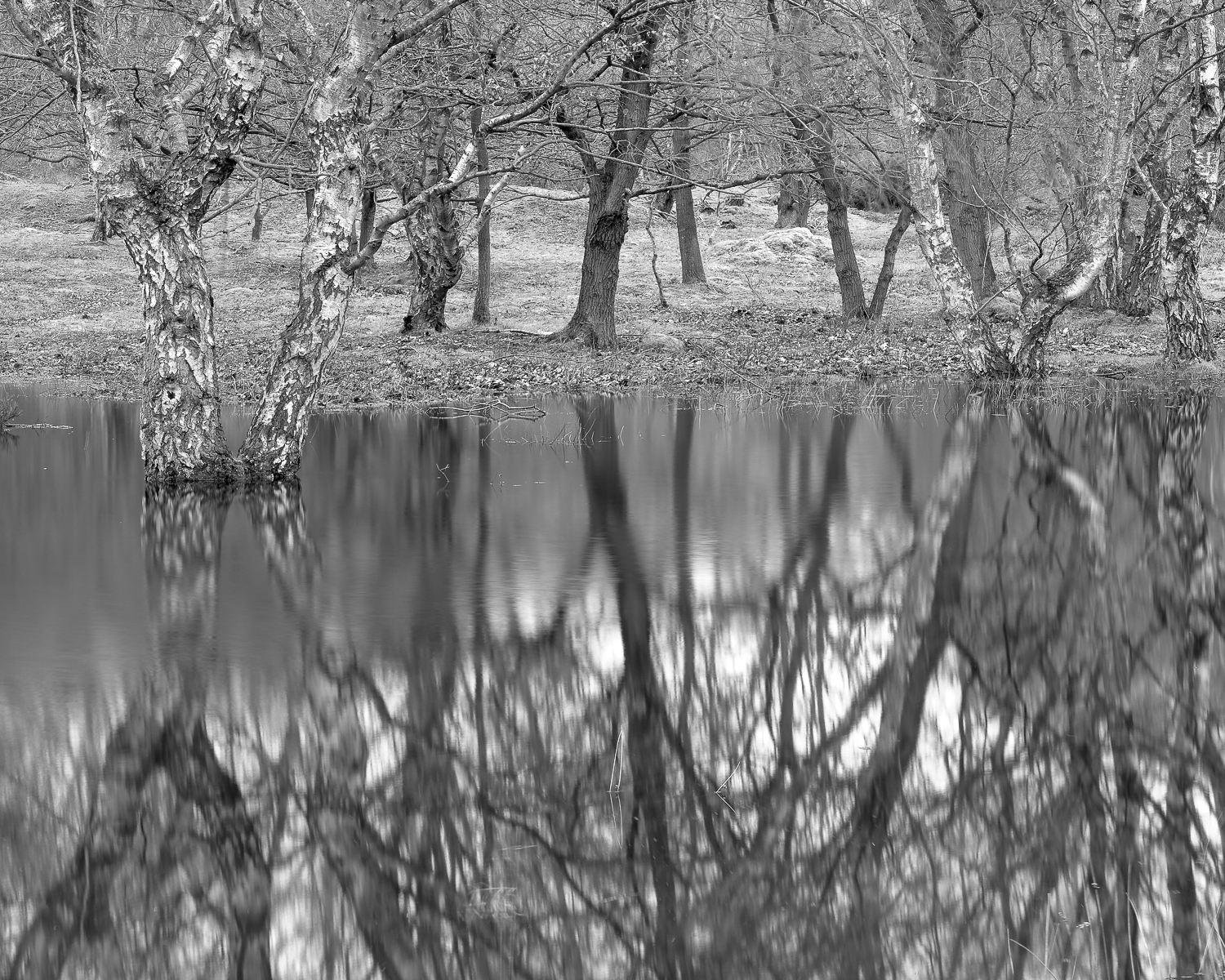S i l v e r   B i r c h   -   N e a r   P i g e o n   P o n d   P l a n t a t i o n.

S c i r y u d a.   S h e r w o o d   F o r e s t,   N o t t i n g h a m s h i r e.

SciryudaO a k.   N e a r   P i g e o n   P o n d   P l a n t a t i o n.

S c i r y u d a.   S h e r w o o d   F o r e s t,   N o t t i n g h a m s h i r e.

Sciryuda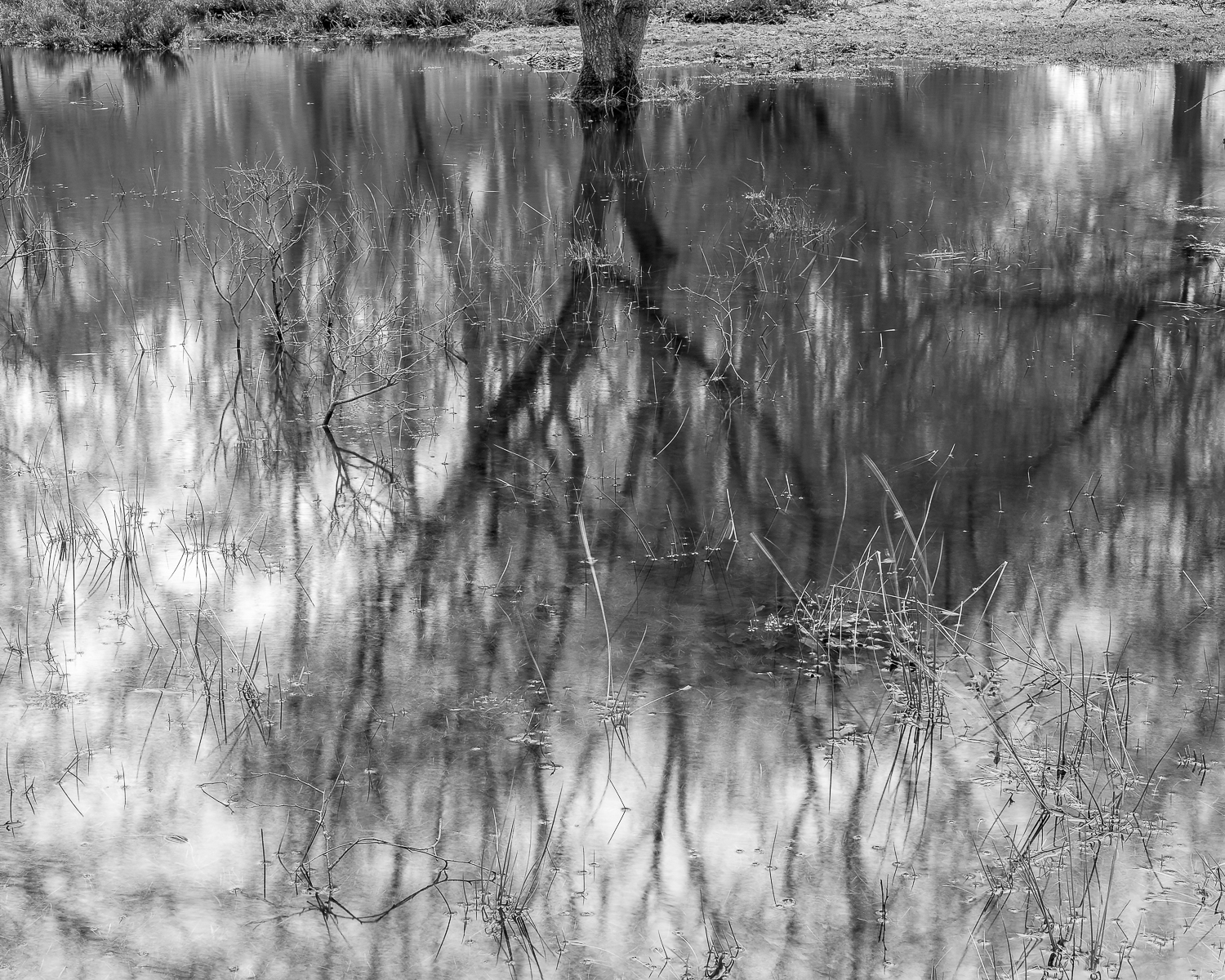O a k.   N e a r   P i g e o n   P o n d   P l a n t a t i o n.

S c i r y u d a,   S h e r w o o d   F o r e s t,   N o t   t   i n g h a m s h i r e.

Sciryuda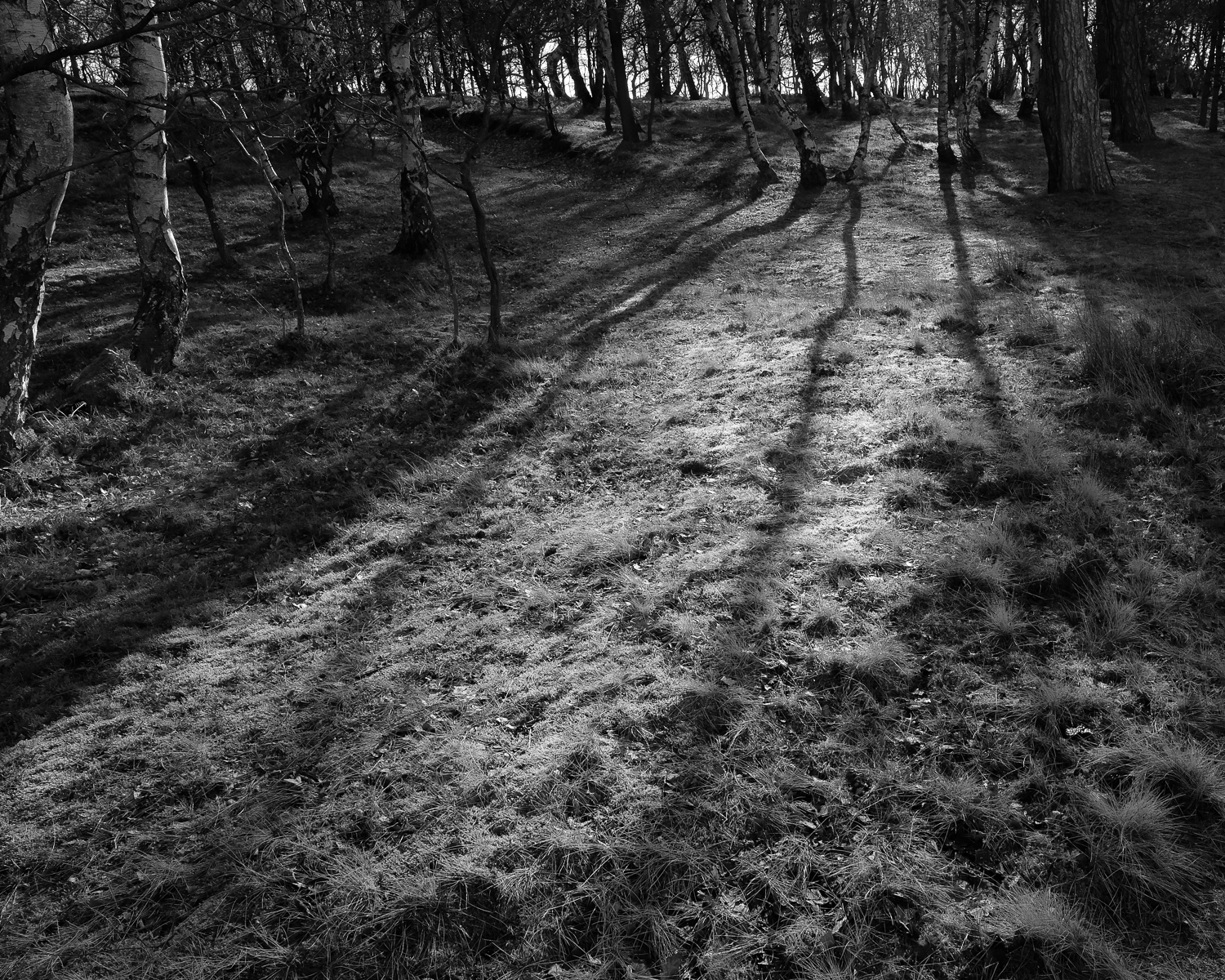C r o w n   H i l l   P l a n t a t i o n,   B u d b y   S o u t h   F o r e s t.

S c i r y u d a,   S h e r w o o d   F o r e s t,   N o t t i n g h a m s h i r e.

Sciryuda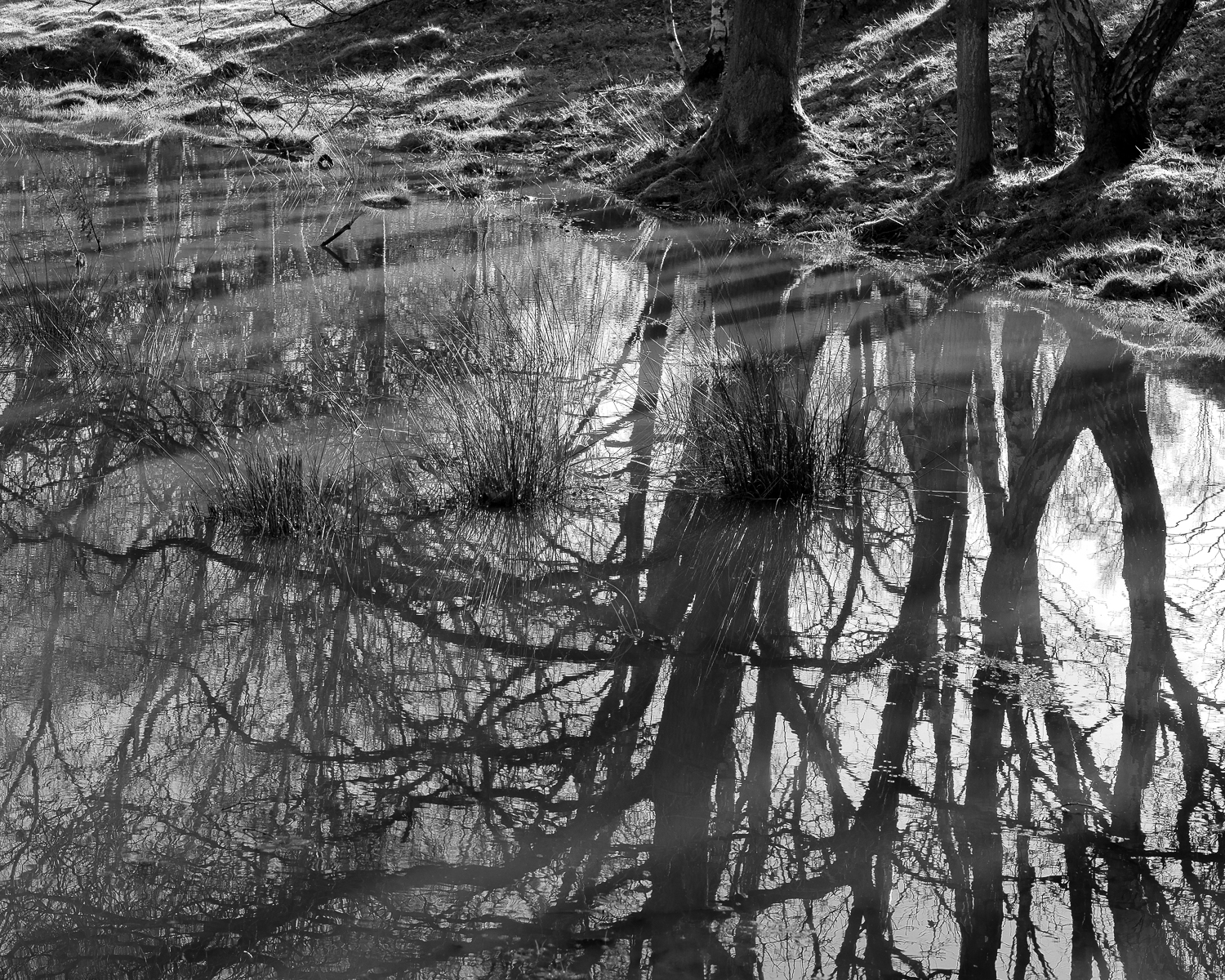B u d b y   S o u t h   F o r e s t

S c i r y u d a.   S h e r w o o d   F o r e s t,   N o t t i n g h a m s h i r e.

Sciryuda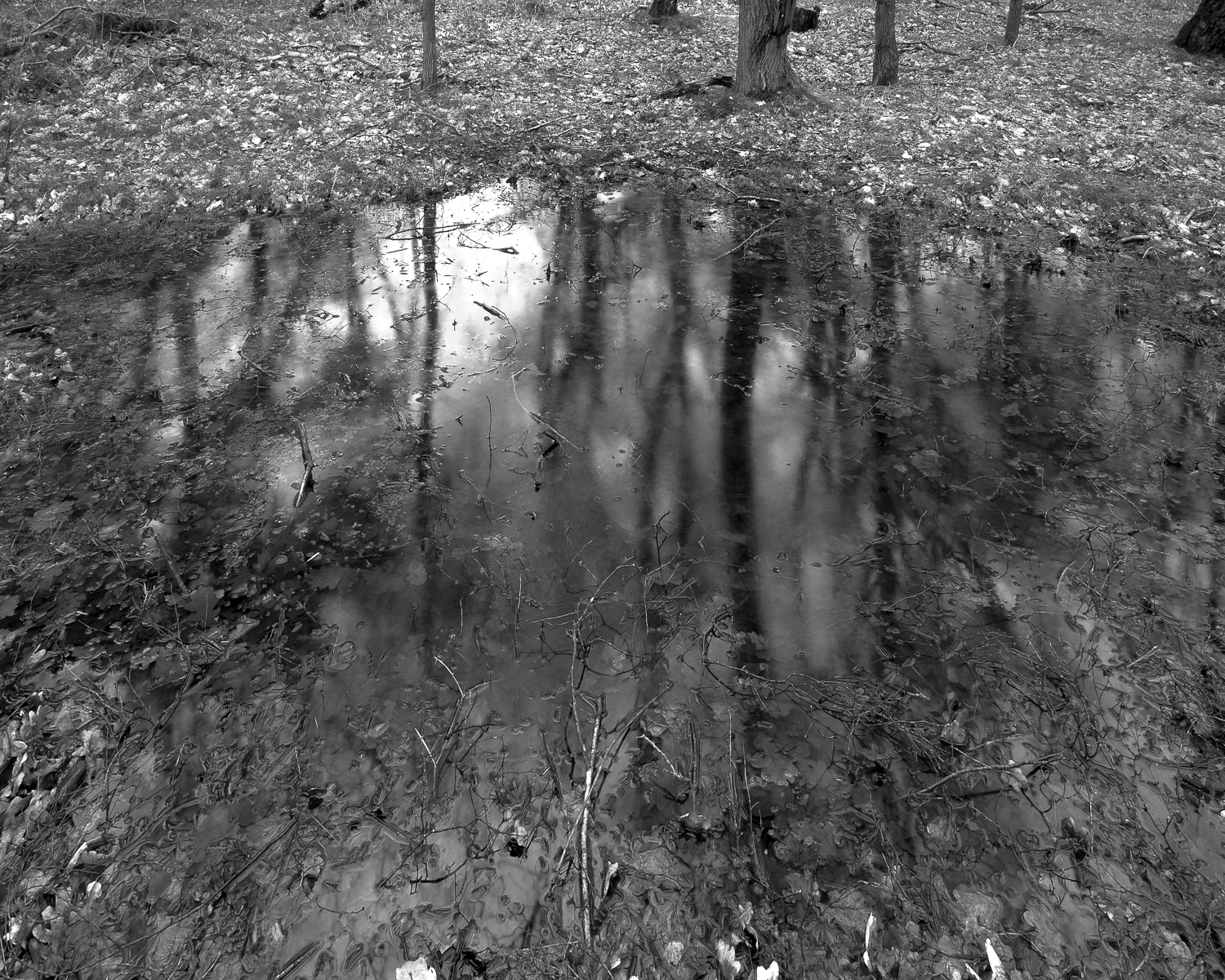P o n d.   C r o w n   H i l l   P l a n t a t i o n.   B u d b y   S o u t h   F o r e s t.

S c i r y u d a,   S h e r w o o d   F o r e s t,   N o t t i n g h a m s h i r e.

Sciryuda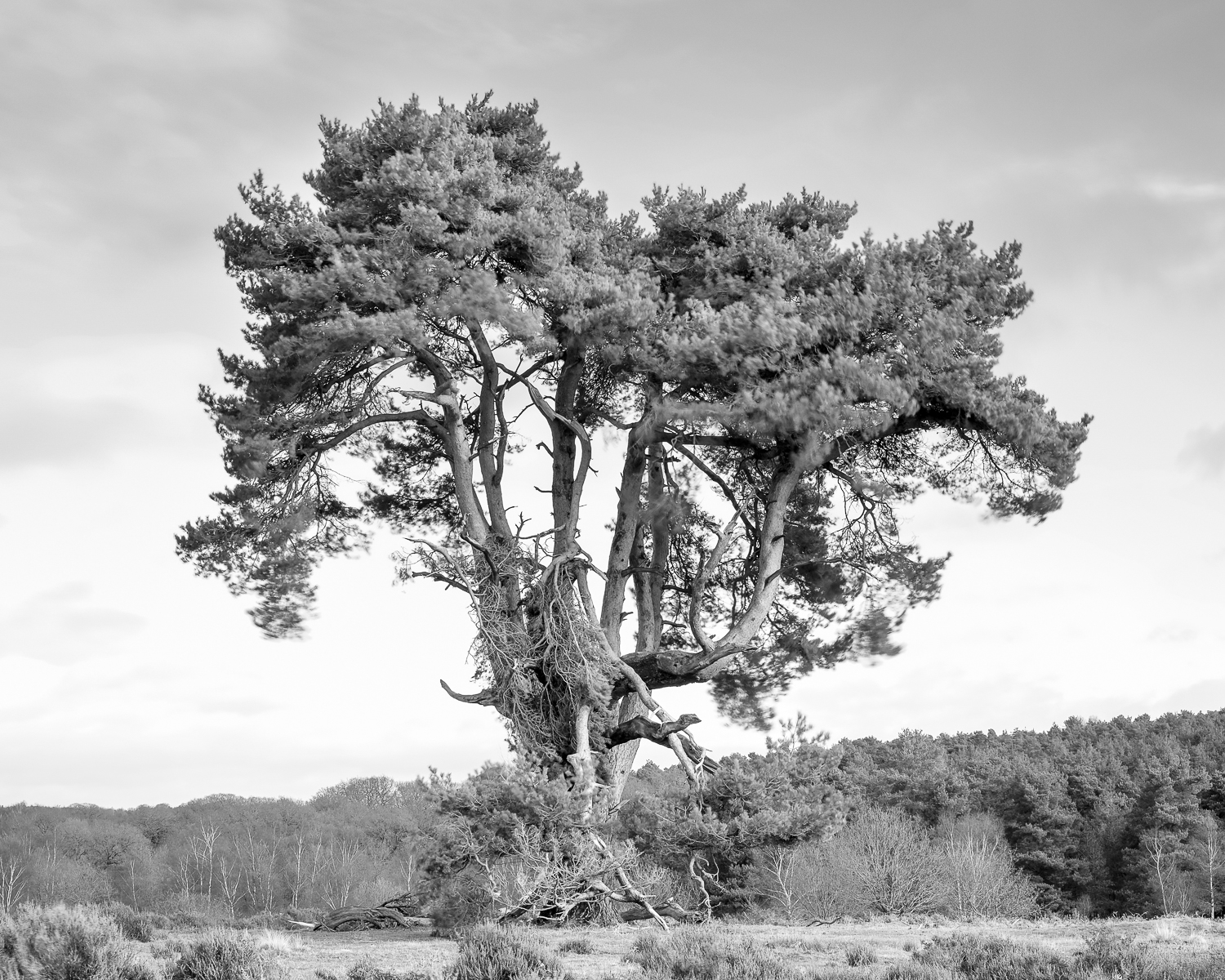S c o t s   P i n e.   B u d b y   S o u t h   F o r e s t.

S c i r y u d a,   S h e r w o o d   F o r e s t,   N o t t i n g h a m s h i r e.

Sciryuda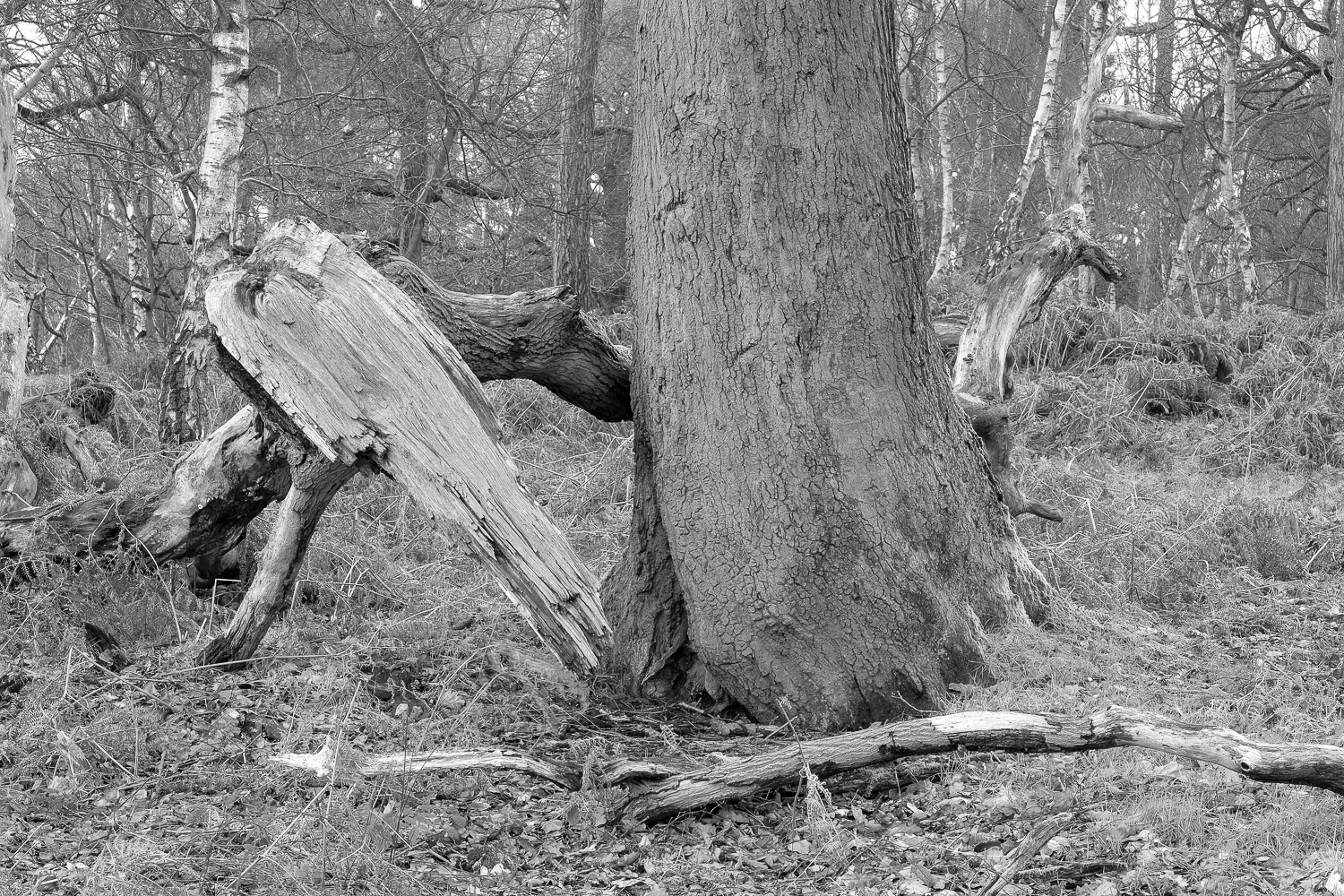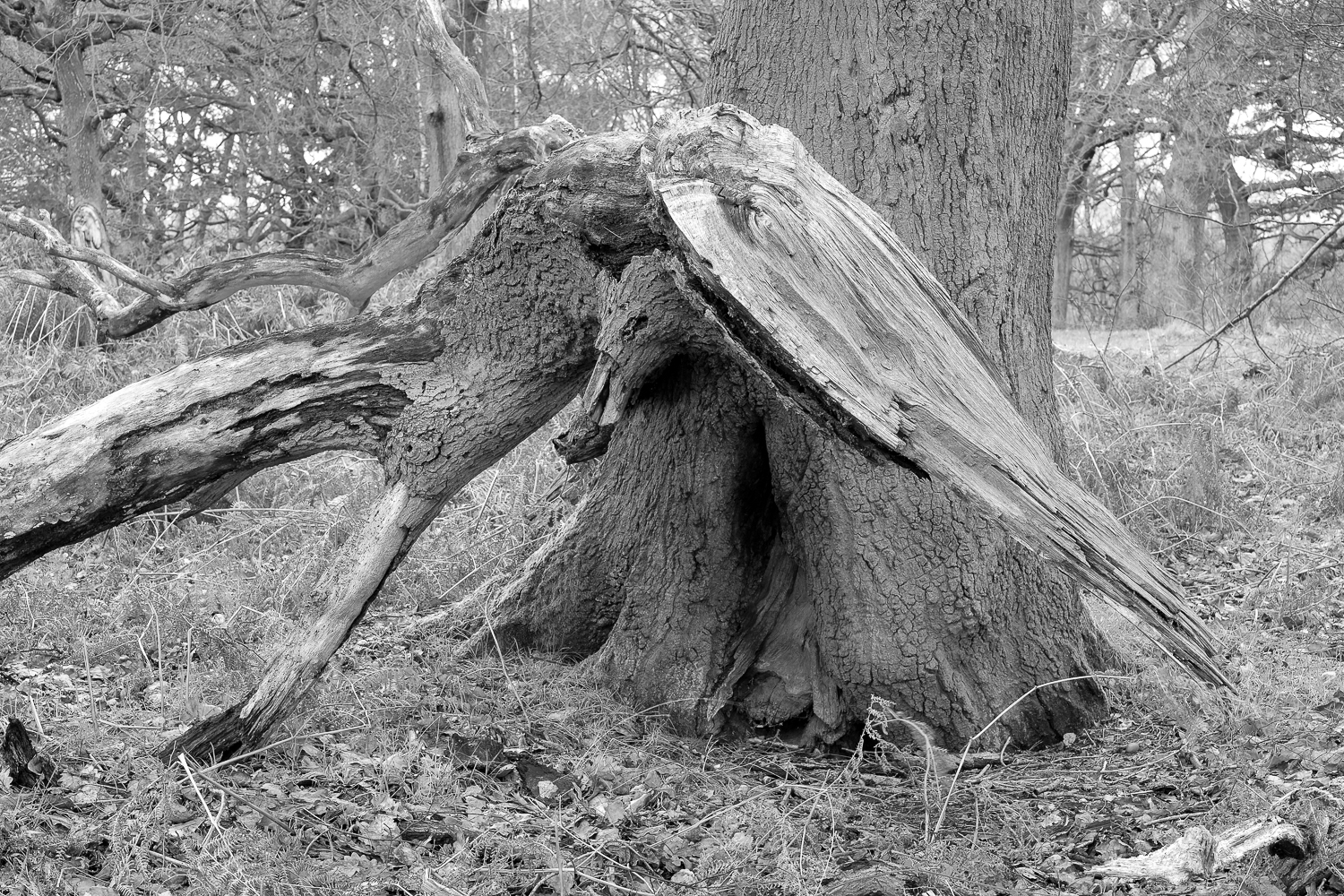S e a r c h i n g.   O a k.   C r o w n   H i l l   P l a n t a t i o n.

S c i r y u d a.   S h e r w o o d   F o r e s t,   N o t t i n g h a m s h i r e.

SciryudaS t o r m.   B u d b y   S o u t h   F o r e s t.

S c i r y u d a.   S h e r w o o d   F o r e s t,   N o t t i n g h a m s h i r e.

Sciryuda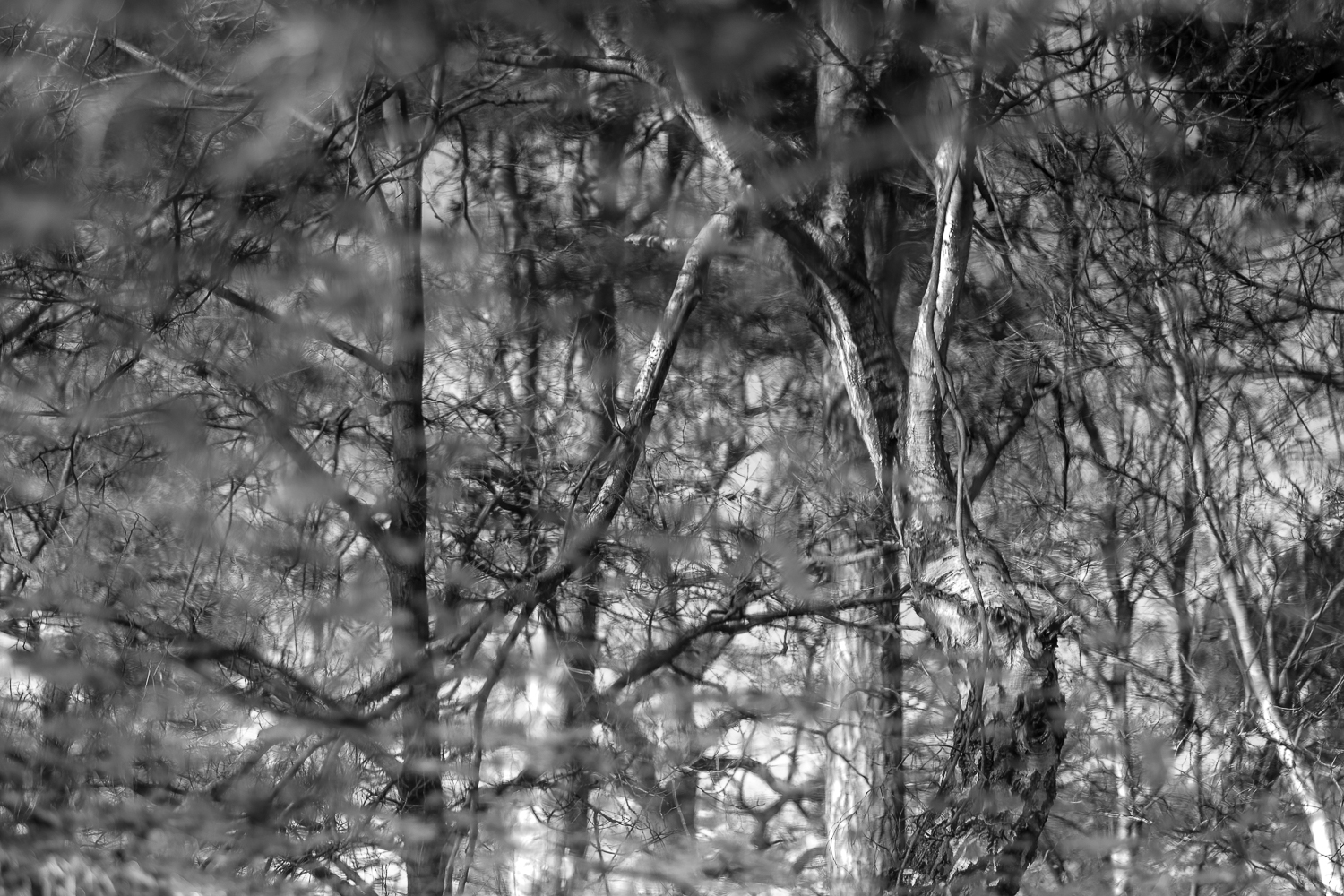B i r c h,   O a k,   P i n e.  W i l d   P o n d.

C r o w n   H i l l   P l a n t a t i o n,   B u d b y   S o u t h   F o r e s t

S c i r y u d a,   S h e r w o o d   F o r e s t,   N o t t i n g h a m s h i r e.

Sciryuda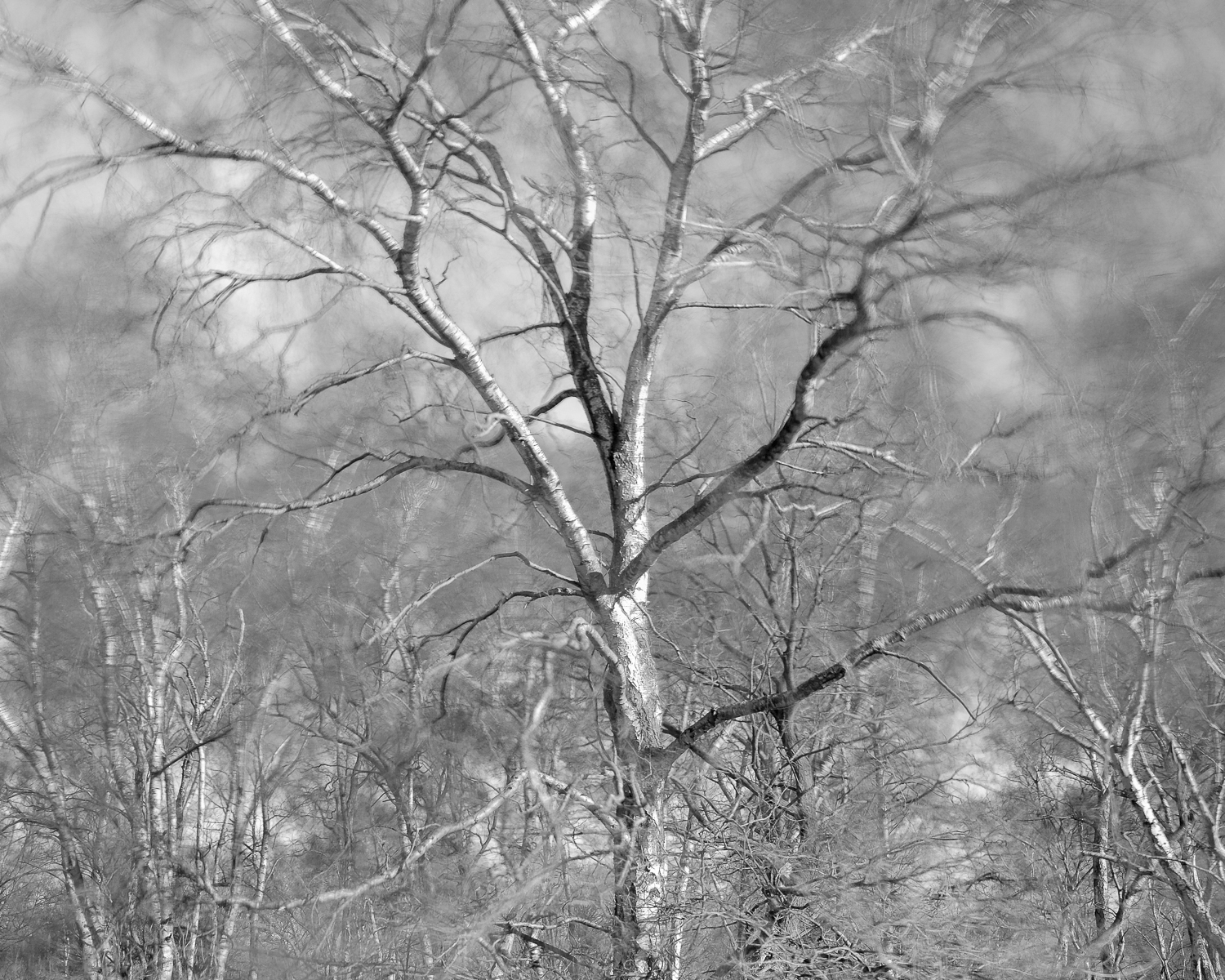S i l v e r   B i r c h   -   ( S t o r m   C i a r a ).   B u r s t h e a r t   H i l l.

S c i r y u d a.   S h e r w o o d   F o r e s t,   N o t t i n g h a m s h i r e.

Sciryuda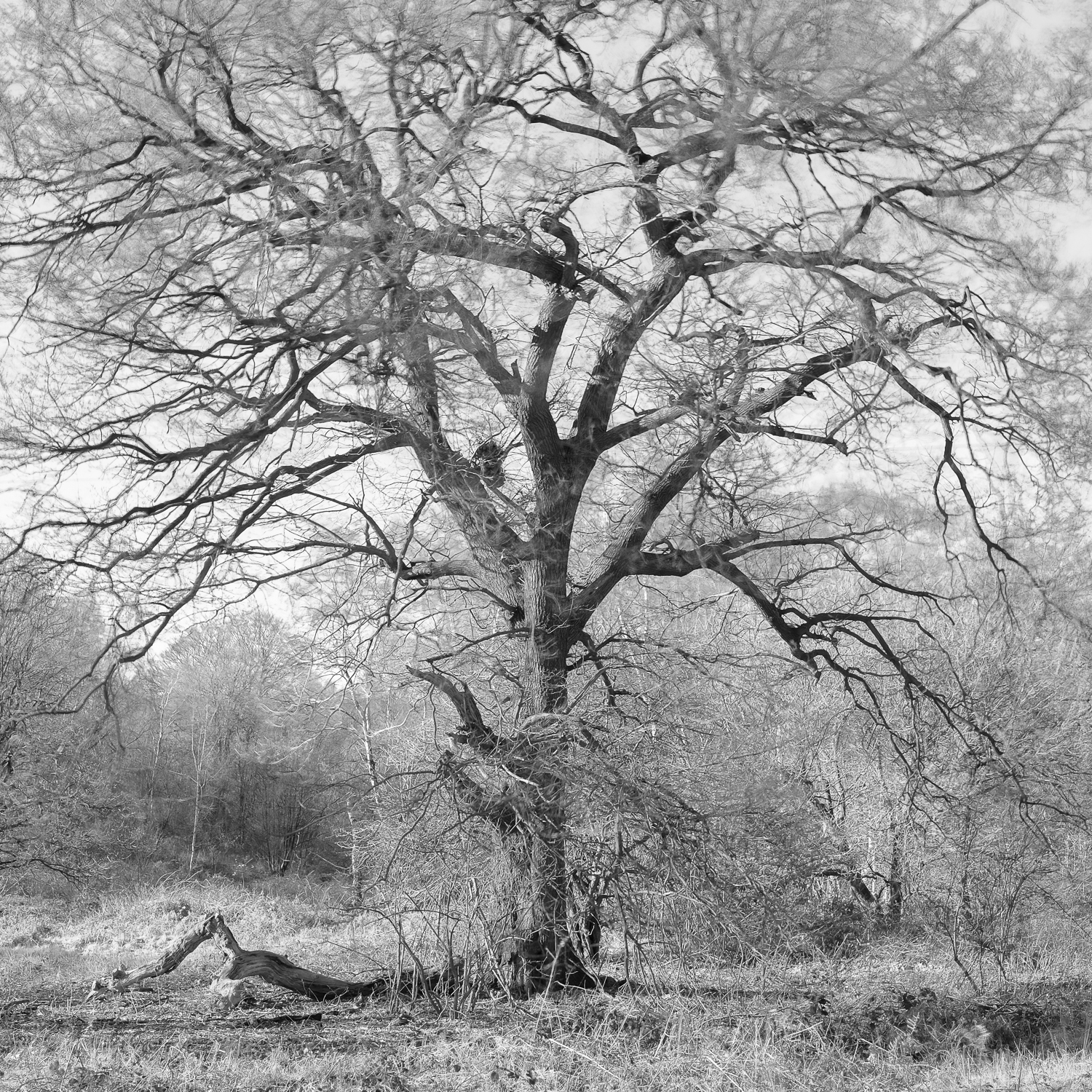O a k.   N e a r   B u r s t h e a r t   H i l l.

S c i r y u d a,   S h e r w o o d   F o r e s t,   N o t t i n g h a m s h i r e.

Sciryuda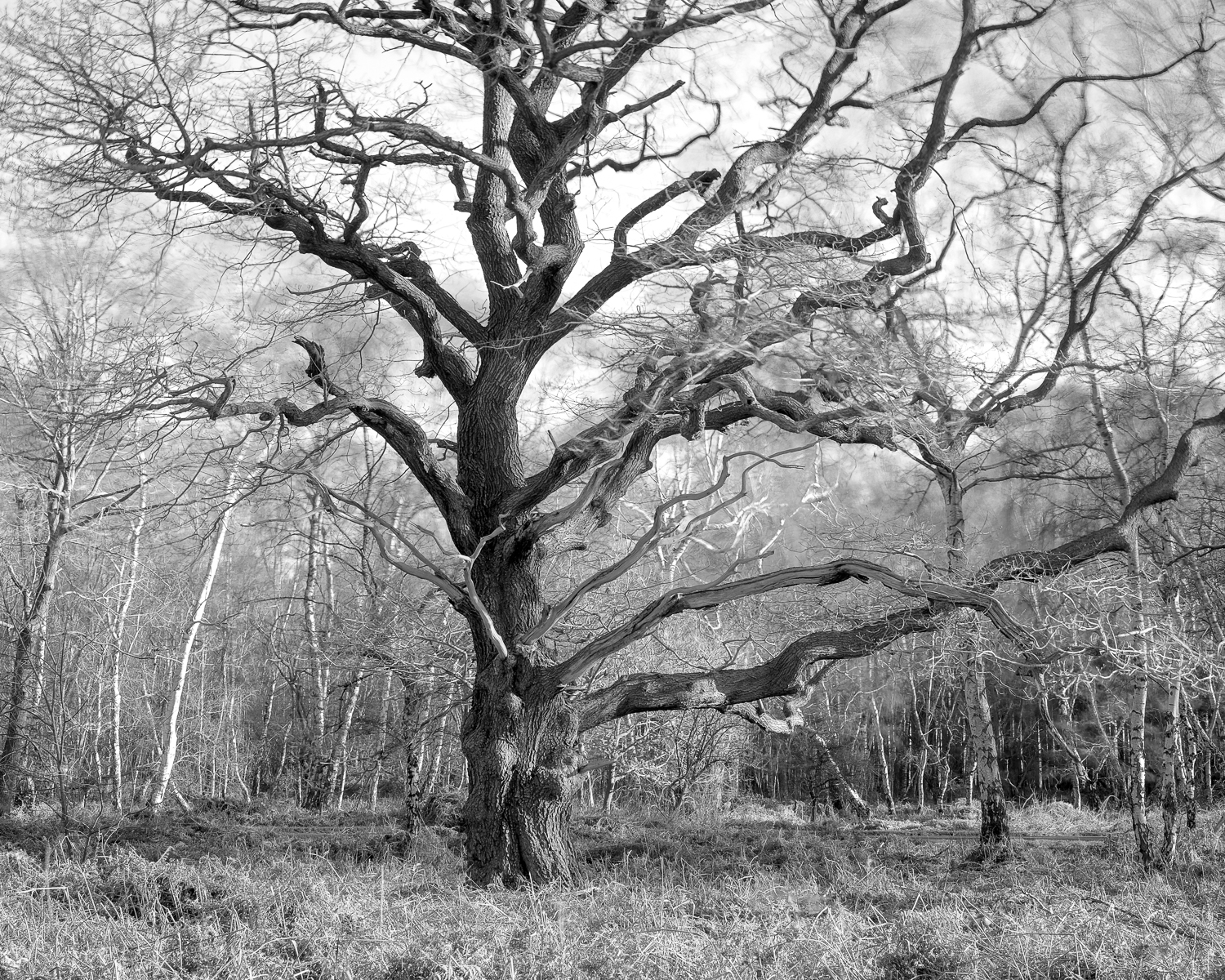O a k.   N e a r   t o   B u r s t h e a r t   H i l l   -   S h e r w o o d   H e a t h

S c i r y u d a,   S h e r w o o d   F o r e s t,   N o t t i n g h a m s h i r e.

Sciryuda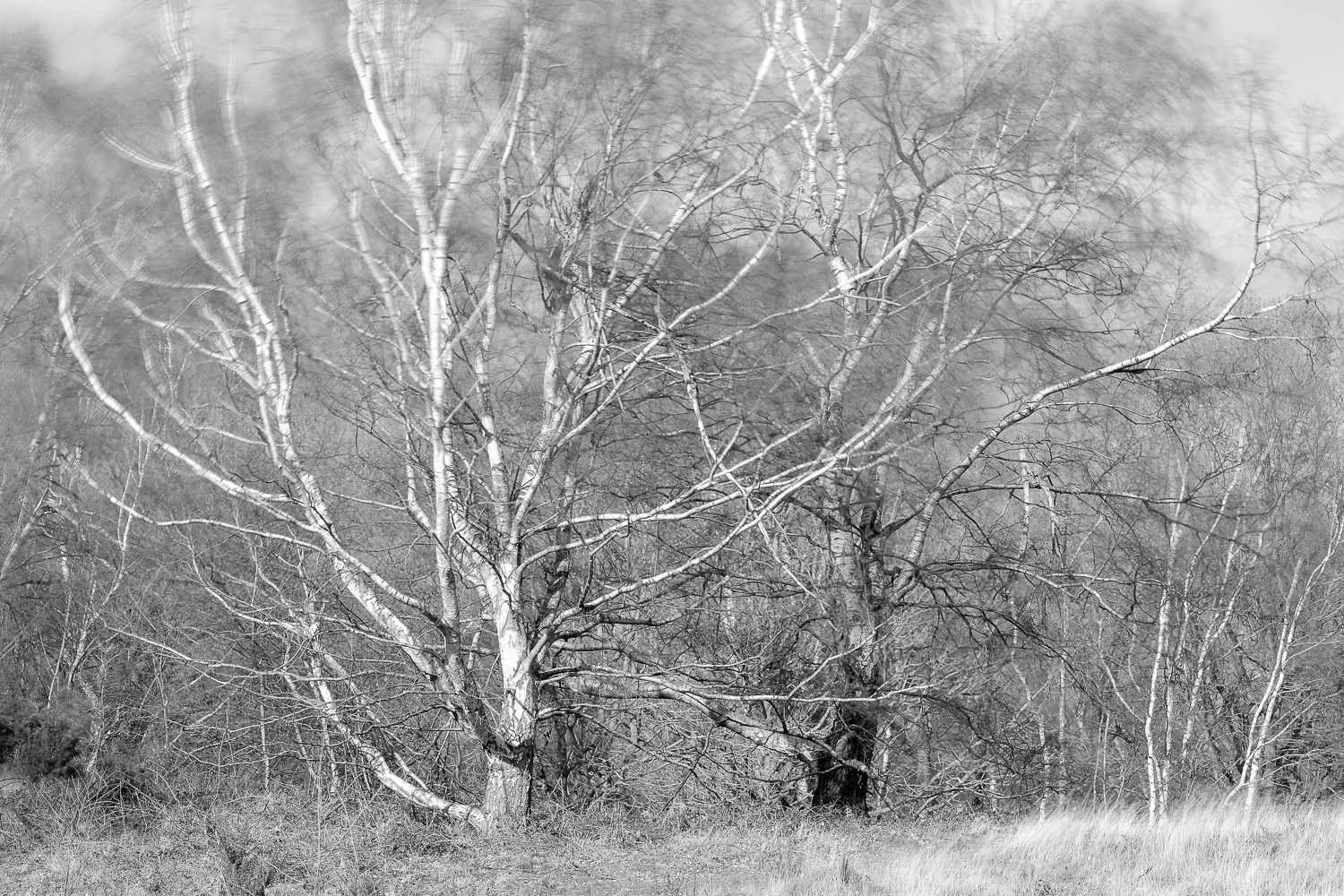S i l v e r   B i r c h e s  -  S h e r w o o d   H e a t h.

S c i r y u d a.   S h e r w o o d   F o r e s t,   N o t t i n g h a m s h i r e.

Sciryuda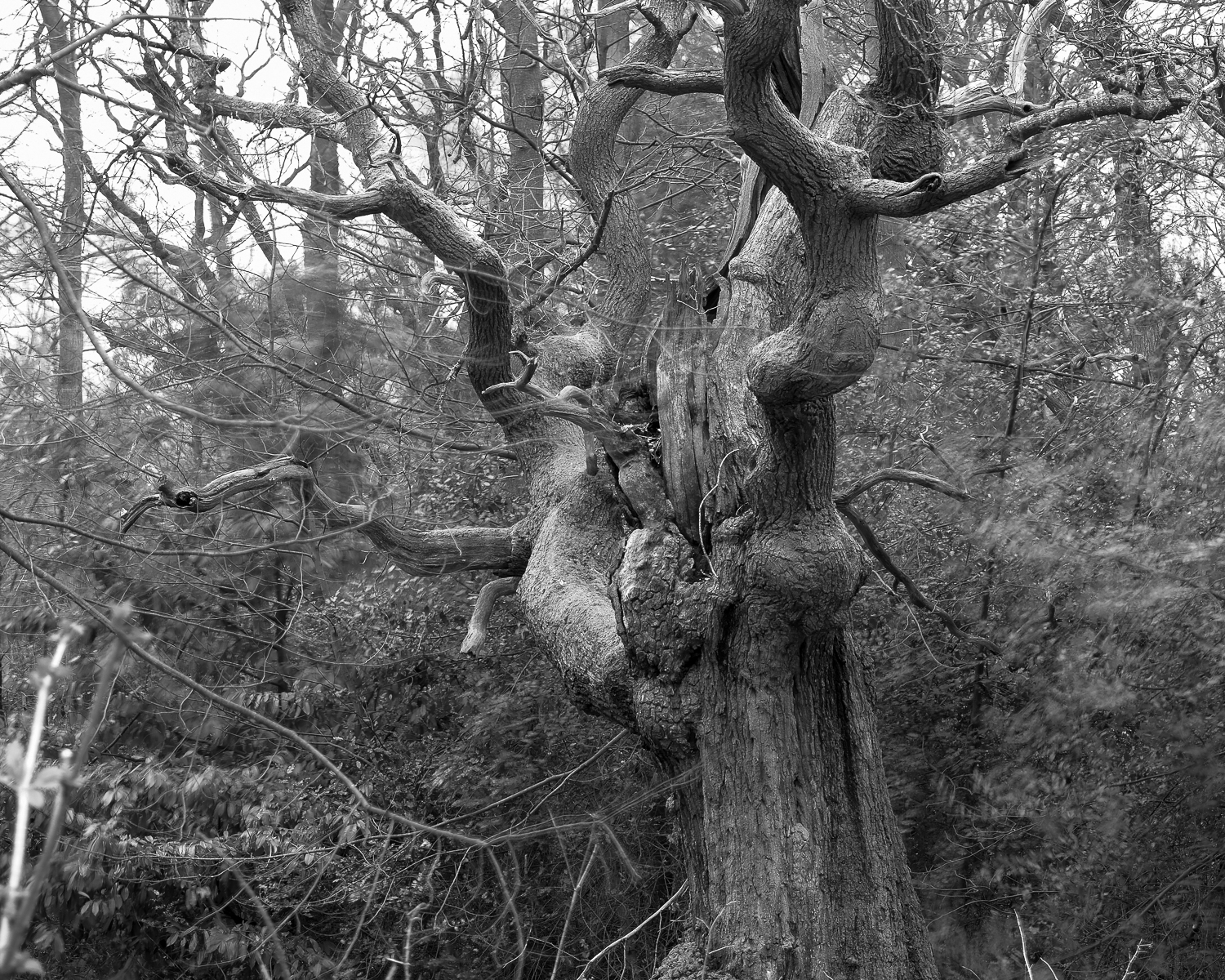A n c i e n t   O a k   -   C a b i n   P l a n t a t i o n

S c i r y u d a,   S h e r w o o d   F o r e s t,   N o t t i n g h a m s h i r e.

Sciryuda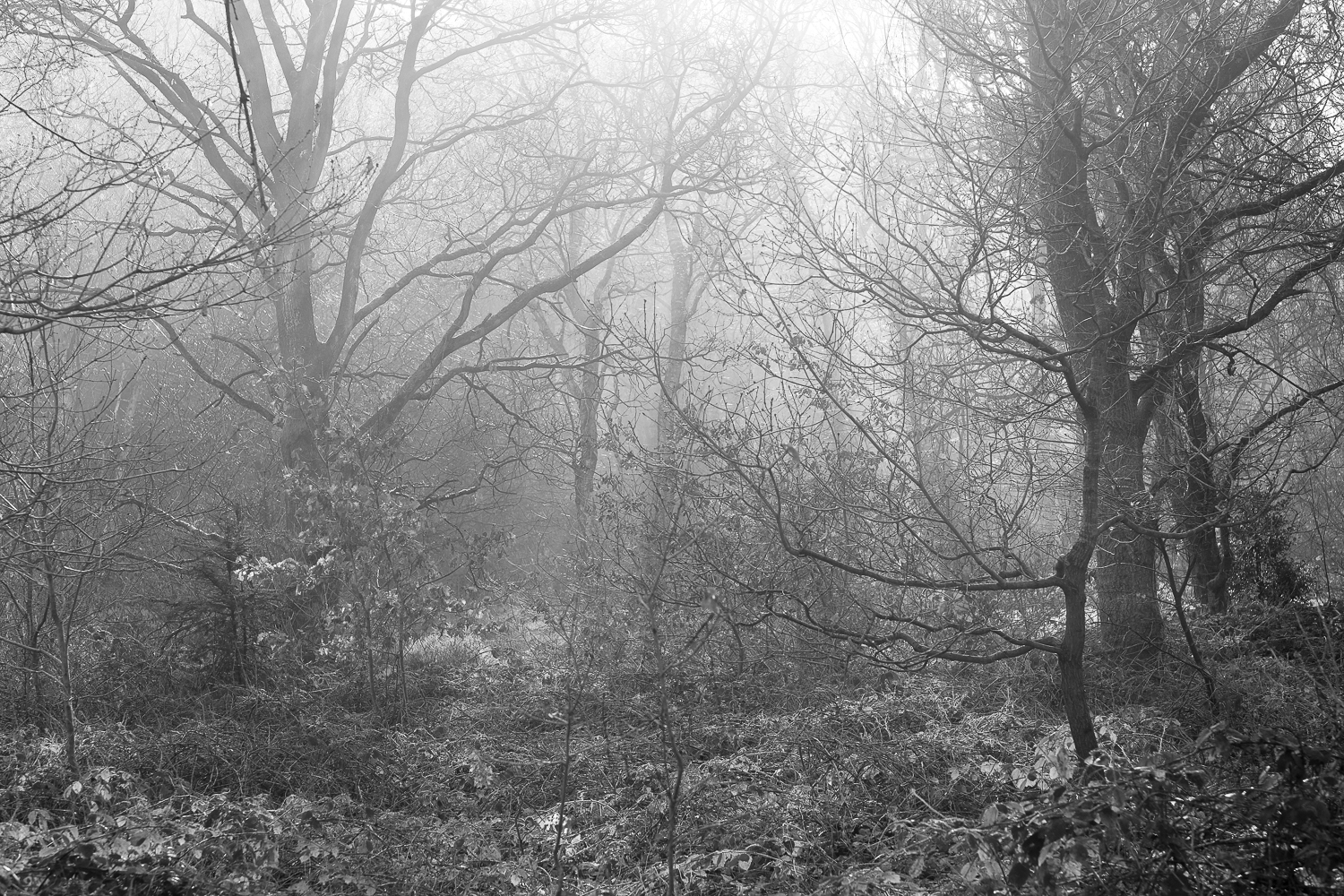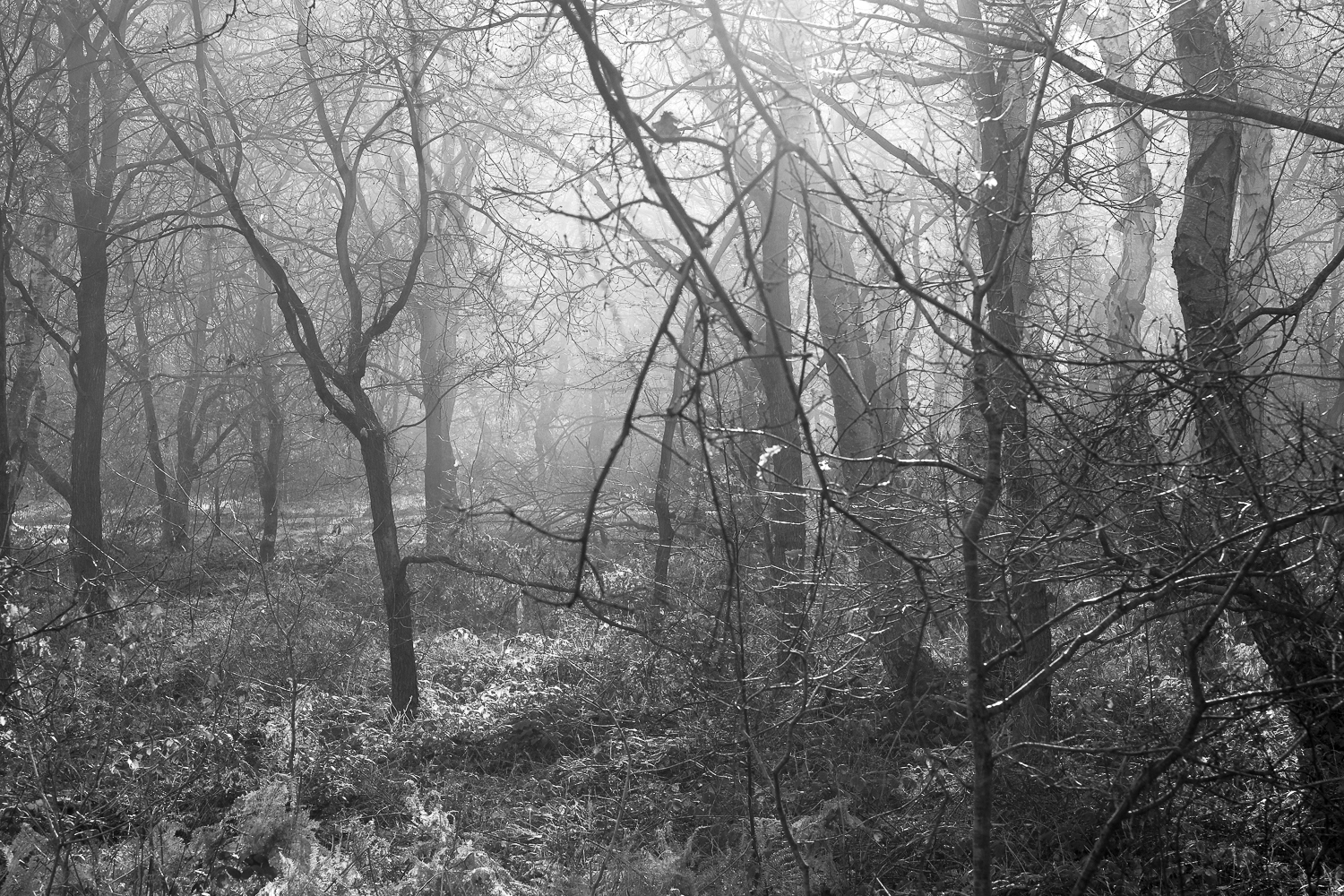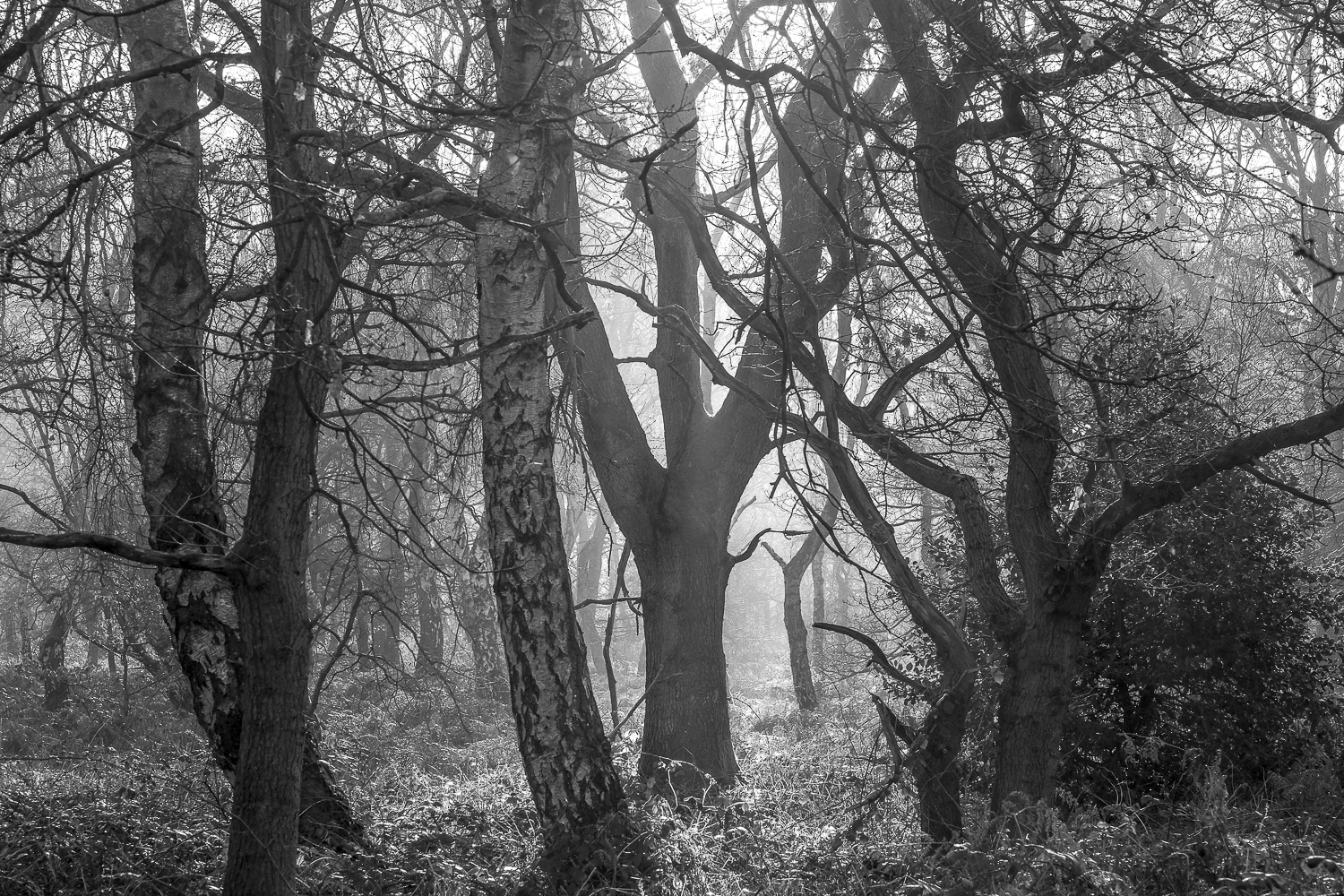Q u e e n   O a k   P l a n t a t i o n.

S c i r y u d a,   S h e r w o o d   F o r e s t,   N o t t i n g h a m s h i r e.

Sciryuda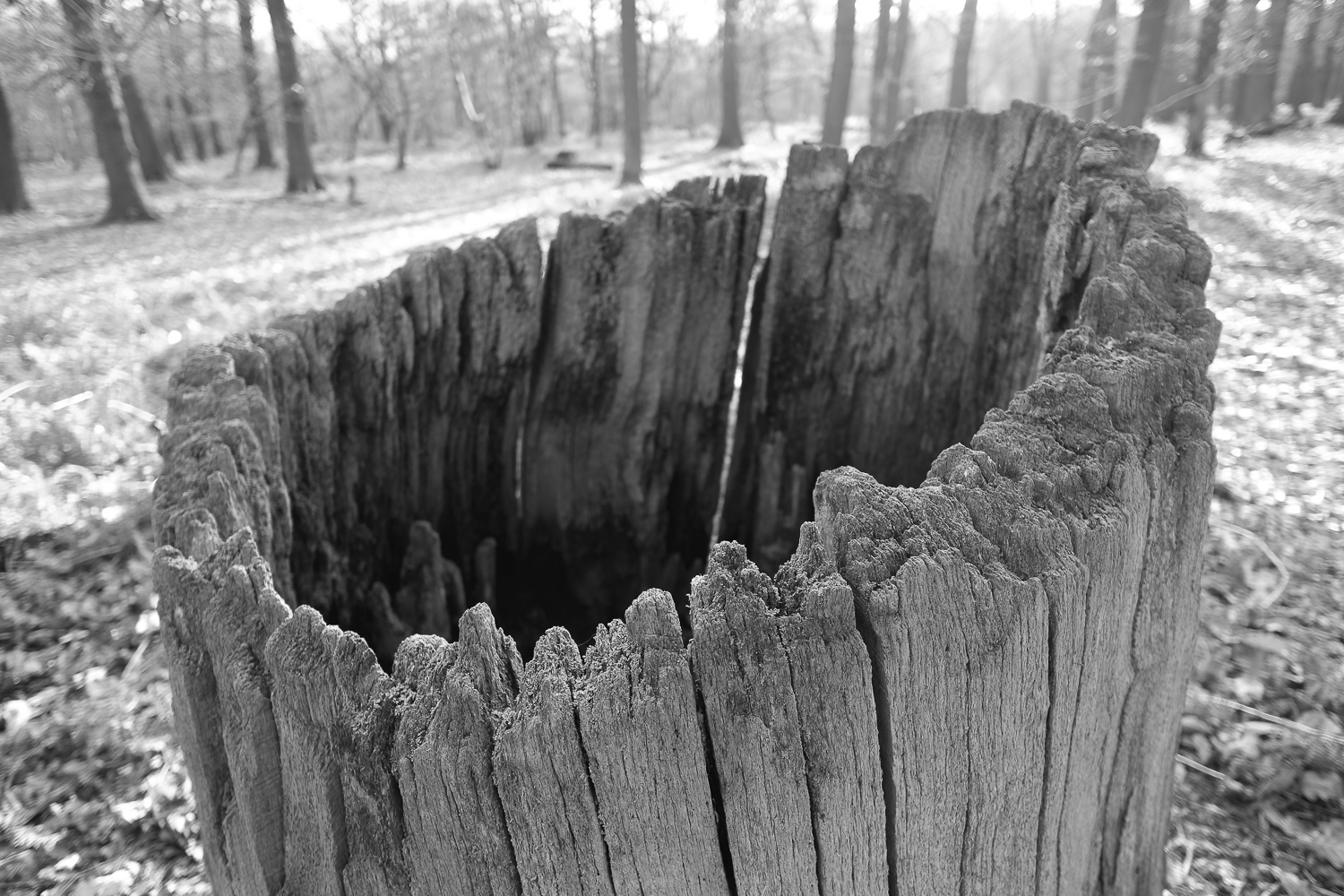S a w n   S t u m p   ( A n c i e n t   O a k )  -  B i r k l a n d s

S c i r y u d a.   S h e r w o o d   F o r e s t,   N o t t i n g h a m s h i r e.